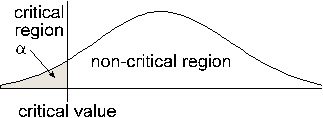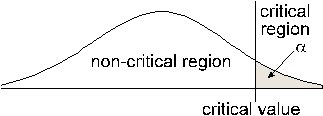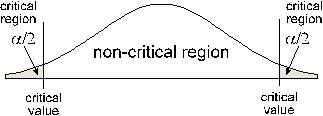Hypothesis Testing

This document will explain how to determine if the test is a left tail, right tail, or two-tail test.

The type of test is determined by the Alternative Hypothesis ( H1 )Left Tailed Test

H1: parameter < value
Notice the inequality points to the left

Decision Rule: Reject H0 if t.s. < c.v.Right Tailed Test

H1: parameter > value
Notice the inequality points to the right

Decision Rule: Reject H0 if t.s. > c.v.Two Tailed Test

H1: parameter not equal value
Another way to write not equal is < or >
Notice the inequality points to both sides

Decision Rule: Reject H0 if t.s. < c.v. (left) or t.s. > c.v. (right)

The decision rule can be summarized as follows:

Reject H0 if the test statistic falls in the critical region

(Reject H0 if the test statistic is more extreme than the critical value)